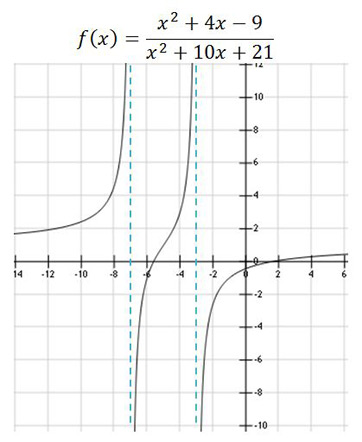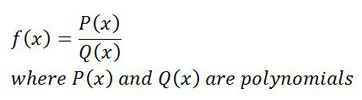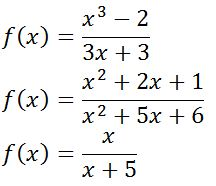# Vertical Asymptotes: Definition & Rules

An error occurred trying to load this video.

Try refreshing the page, or contact customer support.

Coming up next: How to Find the Square Root of a Number

### You're on a roll. Keep up the good work!

Replay
Your next lesson will play in 10 seconds
• 0:00 What Are Vertical Asymptotes?
• 0:30 Rules
• 1:25 Functions
• 2:05 Determining Vertical…
• 3:50 Lesson Summary

Want to watch this again later?

Timeline
Autoplay
Autoplay
Speed

#### Recommended Lessons and Courses for You

Lesson Transcript
Instructor: Yuanxin (Amy) Yang Alcocer

Amy has a master's degree in secondary education and has taught math at a public charter high school.

Vertical asymptotes are interesting mathematical phenomena that occur with certain functions. You will learn where you will see them, what they look like, and how to find them in this lesson.

## What Are Vertical Asymptotes?

Vertical asymptotes are invisible vertical lines that certain functions approach, yet do not cross, when the function is graphed. When you graph some mathematical functions, you will see that the resultant curve avoids certain invisible lines in the graph. No matter what, you can't get the graph to cross those lines. Let me show you what it looks like.The dashed lines have been drawn in to show you where the vertical asymptotes are. Do you see how the graph avoids those areas?

## Rules

There are some rules that vertical asymptotes follow.

1. The graph tends to either positive or negative infinity as it gets closer to the vertical asymptote. Look at the graph and notice how the curve goes either all the way up or all the way down as it nears the asymptote.
2. The distance between the asymptote and the graph tends to zero as the graph gets closer to the asymptote. The graph and the asymptote will seem to almost merge together at the tips, but the curve will never actually touch the asymptote. It is as if the vertical asymptote had a protective field around it preventing anything from touching or crossing it.
3. The graph can approach the vertical asymptote from either direction, from either the right or the left. Look at the graph and see how the graph approaches from both directions. Some functions only approach from only one direction, but like our function, others can approach from both.

## Functions

The function that we graphed is somewhat complex and is called a rational function. In this lesson, we will focus on the vertical asymptotes of rational functions. There are other functions that also produce vertical asymptotes, but rational functions are the most common.

A rational function is a function whose numerator and denominator are made up of polynomials. The general form of a rational function is the following.Here are some examples of rational functions.All of the above are fractions where both the numerator and denominator are polynomials. Because of this, this type of function makes it easy for you to find the vertical asymptotes.

## Determining Vertical Asymptotes

To determine the vertical asymptotes of a rational function, all you need to do is to set the denominator equal to zero and solve. Vertical asymptotes occur where the denominator is zero. Remember, division by zero is a no-no. Because you can't have division by zero, the resultant graph thus avoids those areas.

To unlock this lesson you must be a Study.com Member.

### Register to view this lesson

Are you a student or a teacher?

### Unlock Your Education

#### See for yourself why 30 million people use Study.com

##### Become a Study.com member and start learning now.
Back
What teachers are saying about Study.com

### Earning College Credit

Did you know… We have over 160 college courses that prepare you to earn credit by exam that is accepted by over 1,500 colleges and universities. You can test out of the first two years of college and save thousands off your degree. Anyone can earn credit-by-exam regardless of age or education level.